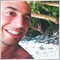# problem with #property strict32

Hello

I have a problem with the following coding.

I know the problem is because of using the following code :

`#property strict`

When I use it, I have the  error : 2019.11.16 12:03:11.915 2017.01.02 00:00:00  S_R EURUSD,H1: array out of range (75,43)
Can you help me solve the problem?

thanks

```#property copyright ""
#property strict
#property indicator_chart_window
#property indicator_buffers 4
#property indicator_color1 Yellow
#property indicator_color2 Yellow

//---- input parameters

//---- buffers
double ExtMapBuffer1[];
double ExtMapBuffer2[];
double ExtMapBuffer3[];

int K;
//+------------------------------------------------------------------+
//| Custom indicator initialization function                         |
//+------------------------------------------------------------------+
int init()
{
//---- indicators
SetIndexStyle(0,DRAW_ARROW,STYLE_DOT,1,Yellow);
SetIndexDrawBegin(0,K-1);
SetIndexBuffer(0,ExtMapBuffer1);
SetIndexLabel(0,"Resistance M15");
SetIndexArrow(0, 158);
SetIndexStyle(1,DRAW_ARROW,STYLE_DOT,1,Yellow);
SetIndexDrawBegin(1,K-1);
SetIndexBuffer(1,ExtMapBuffer2);
SetIndexLabel(1,"Support M15");
SetIndexArrow(1, 158);

//----
return(0);
}
//+------------------------------------------------------------------+
//| Custor indicator deinitialization function                       |
//+------------------------------------------------------------------+
int deinit()
{
return(0);
}
//------------------------------------------------------------------
bool Fractal (string M,int P, int shift)
{
if (Period()>P) return(0);
P=P/Period()*2+MathCeil(P/Period()/2);
if (shift<P)return(0);
if (shift>Bars-P)return(0);
for (int i=1;i<=P;i++)
{
if (M=="U")
{
if (High[shift+i]>High[shift])return(0);
if (High[shift-i]>=High[shift])return(0);
}
if (M=="L")
{
if (Low[shift+i]<Low[shift])return(0);
if (Low[shift-i]<=Low[shift])return(0);
}
}
return(1);
}
//------------------------------------------------------------------
int start()
{
int  M15=15;
K=Bars;
while(K>=0)
{
if (Fractal("U",M15,K)==1) ExtMapBuffer1[K]=High[K];
else ExtMapBuffer1[K]=ExtMapBuffer1[K+1];
if (Fractal("L",M15,K)==1) ExtMapBuffer2[K]=Low[K];
else ExtMapBuffer2[K]=ExtMapBuffer2[K+1];

K--;
}

return(0);
}```3768

You are trying to assign to ExtMapBuffer1[K] the value of K+1. At the beginning K is equal to Bars, and K+1 will be Bars+1. The array of an indicator cannot be bigger than bars.

Try to change the code in this way

```K=Bars-1;
```

Please, consider the use of IndicatorCounted() in order to avoid indicator to recalculate ALL bars for each new tick, it will be very resources heavy.20210

You should stop using the old event handlers and IndicatorCounted() and start using the new ones.
Event Handling Functions - Functions - Language Basics - MQL4 Reference
How to do your lookbacks correctly.